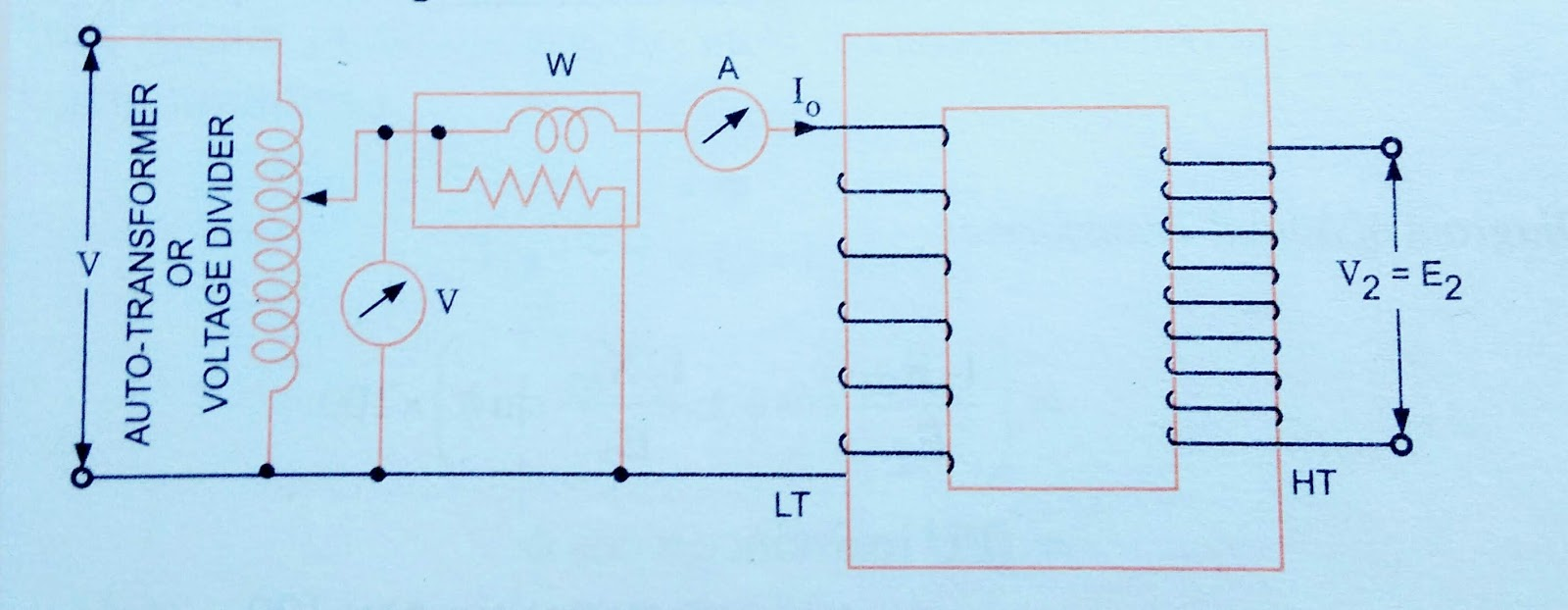### Ticker

6/recent/ticker-posts

# How to understood of Transformer Test ????

TRANSFORMER TEST

Open Circuit or No load Test

This test is performed to determine core or iron loss Pi and no load Current I0. This test is helpful in determination of magnetizing components Im, energy component Ie and no load Resistance R0 being given as Vi/Ie and no-load Reactance given as Vi/Im.
In this test secondary ( usually High Voltage) winding is left open, all metering instruments ( ammeter, voltmeter and wattmeter ) are connected on primary ( low voltage ) wind winding, as illustrated in fig. (a).CKT Diagram for Open-circuit Test

The open-circuit test gives enough data to compute the equivalent Circuit constants R0, X0, no-load Power factor CosΦ0, no-load current I0 and no-load power loss ( iron loss ) of a transformer.
Iron loss, Pi = input power on no load = P0 watts ( say)
No - load Current = I0 amps.
Applied Voltage to primary = V1 volts

P0
Angle of lag, Φ0 = Cos^-1 --------    ___(1)
V1 I0

P0
Ie = I0 CosΦ0 = -------    ____(2)
V1

Im = √ i0^2 - ie^2   ____(3)

Equivalent Circuit parameter,

V1         V1^2
R0 = -------- = -----------     ____(4)
Ie           P0
Equivalent Circuit parameter,

V1                  V1
X0 = -------  =.        --------------      ___(5)
Im         √ I0^2 - Ie^2

Causion. Since no-load Current I0 is very small, therefore, pressure Coil of wattmeter and the voltmeter should be connected such that Current taken by them should not flow through the Current Coil of the wattmeter.

Short Circuit Test oR Impedance Test.

This test is performed to determine the full-load copper loss and equivalent resistance and Reactance referred to Secondary side.
In this test, the terminal of the secondary windings are short circuited, all meters ( ammeter, voltmeter, and wattmeter) rae connected on primary side and a low voltage , usually 5 to 10 % of normal rated primary, as shown in fig.(b)

b) Circuit Diagram for short-                                                  circuit test

The applied voltage to the primary, as Vs is Gradually increase till the ammeter A indicates the full-load Current of the side in which it is connected. The reading Ws of the wattmeter gives total Copper loss [ iron losses being negligible due to very low voltage resulting in very small flux Linking with the core ) at full load.
Let the ammeter reading be Is.

Full-load copper loss, Pc = Is^2 R eq = Ws
---------(6)

Equivalent Resistance,

Ws
R eq = -------  ______( 7 )
Is^2
Vs
Equivalent impedance, Z eq = -------  ___(8)
I s
Equivalent Reactance,
X eq = √ Z eq^2 - R eq^2 ______( 9 )

If you get knowledge of Electrical Machine so click the